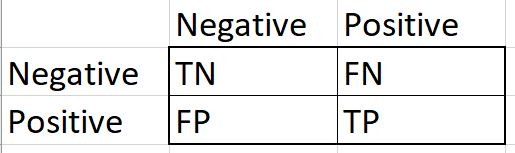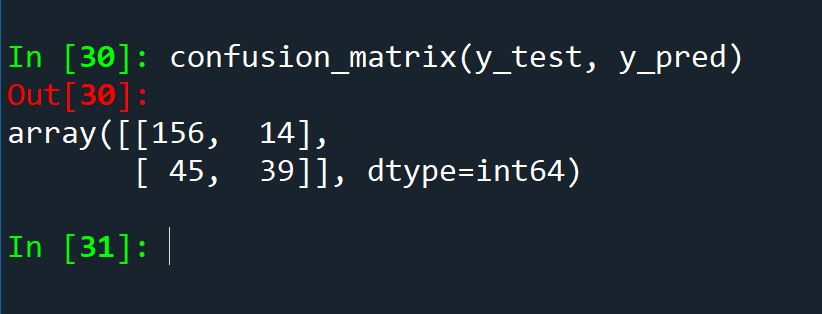# Python: Confusion Matrix

## What is a confusion matrix?

A confusion matrix is a supervised machine learning evaluation tool that provides more insight into the overall effectiveness of a machine learning classifier. Unlike a simple accuracy metric, which is calculated by dividing the number of correctly predicted records by the total number of records, confusion matrices return 4 unique metrics for you to work with.

While I am not saying accuracy is always misleading, there are times, especially when working with examples of imbalanced data,  that accuracy can be all but useless.

Let’s consider credit card fraud. It is not uncommon that given a list of credit card transactions, that a fraud event might make up a little as 1 in 10,000 records. This is referred to a severely imbalanced data.  Now imaging a simple machine learning classifier running through that data and simply labeling everything as not fraudulent. When you checked the accuracy, it would come back as 99.99% accurate. Sounds great right? Except you missed the fraud event, the only reason to try to create the model in the first place.

A confusion matrix will show you more details, letting you know that you completely missed the fraud event. Instead of a single number result, a confusion matrix provides you will 4 metrics to evaluate. (note: the minority class – (in the case of fraud – the fraudulent events) – are labeled positive by confusion matrices. So a non-fraud event is a negative. This is not a judgement between the classes, only a naming convention)

TP = true positive – minority class (fraud) is correctly predicted as positive

FP = false positive – majority class (not fraud) is incorrectly predicted

FN = false negative – minority class (fraud) incorrectly predicted

TN = true negative – majority class (not fraud) correctly predicted

In matrix form:To run a confusion matrix in Python, Sklearn provides a method called confusion_matrix(y_test, y_pred)

y_test = actual results from the test data set

y_pred = predictions made by model on test data set

so in a pseudocode example:

```model.fit(X,y)
y_pred = model.predict(X_test)```

If this is at all confusing, refer to my Python SVM lesson where I create the training and testing set and run a confusion matrix (Python: Support Vector Machine (SVM))

To run a confusion matrix in Python, first run a model, then run predictions (as shown above) and then follow the code below:

```from sklearn.metrics import confusion_matrix
confusion_matrix(y_test, y_pred)```

Output looks like this:Now, if you want to capture the TP, TN, FP, FN into individual variables to work with, you can add the ravel() function to your confusion matrix:

`TN,FP,FN,TP = confusion_matrix(y_test, y_pred).ravel()`

Thank you for taking the time to read this, and good luck on your analytics journey.

# Python: Support Vector Machine (SVM)

## Support Vector Machine (SVM):

A Support Vector Machine, or SVM, is a popular binary classifier machine learning algorithm. For those who may not know, a binary classifier is a predictive tool that returns one of two values as the result, (YES – NO), (TRUE – FALSE), (1 – 0).  Think of it as a simple decision maker:

Should this applicant be accepted to college? (Yes – No)

Is this credit card transaction fraudulent? (Yes – No)

An SVM predictive model is built by feeding a labeled data set to the algorithm, making this a supervised machine learning model. Remember, when the training data contains the answer you are looking for, you are using a supervised learning model. The goal, of course, of a supervised learning model is that once built, you can feed the model new data which you do not know the answer to, and the model will give you the answer.

## Brief explanation of an SVM:

An SVM is a discriminative classifier. It is actually an adaptation of a previously designed classifier called perceptron. (The perceptron algorithm also helped to inform the development of artificial neural networks).

The SVM works by finding the optimal hyperplane that can be used to discriminate between classes in the data set. (Classes refers to the label or “answer” column of each record.  The true/false, yes/no column in a binary set). When considering a two dimensional model, the hyperplane simply becomes a line that divides to the classes of data.

The hyperplane (or line in 2 dimensions) is informed by what are known as Support Vectors. A record from the data set is converted into a vector when fed through the algorithm (this is where a basic understanding of linear algebra comes in handy). Vectors (data records) closest to the decision boundary are called Support Vectors. It is on either side of this decision boundary that a vector is labeled by the classifier.

The focus on the support vectors and where they deem the decision boundary to be, is what informs the SVM as to where to place the optimal hyperplane. It is this focus on the support vectors as opposed to the data set as a whole, that gives SVM an advantage over a simple learner like a linear regression, when dealing with complex data sets.

## Coding Exercise:

Libraries needed:

sklearn

pandas

This is the main reason I recommend the Anaconda distribution of Python, because it comes prepackaged with the most popular data science libraries.

```from sklearn.model_selection import train_test_split
from sklearn.svm import SVC
from sklearn.metrics import metrics
from sklearn.metrics import confusion_matrix
import pandas as pd```

Next, let’s look at the data set. This is the Pima Indians Diabetes data set. It is a publicly available data set consisting of 768 records. Columns are as follows:

1. Number of times pregnant.
2. Plasma glucose concentration a 2 hours in an oral glucose tolerance test.
3. Diastolic blood pressure (mm Hg).
4. Triceps skinfold thickness (mm).
5. 2-Hour serum insulin (mu U/ml).
6. Body mass index (weight in kg/(height in m)^2).
7. Diabetes pedigree function.
8. Age (years).
9. Class variable (0 or 1).

pima_indians

now look at the data:Now keep in mind, class is our target. That is what we want to predict.

So let us start by separating the target class.

We use the pandas command .pop() to remove the Class column to the y variable, and the remained of the dataframe is now in the X

Let’s now split the data into training and test sets:

`X_train, X_test, y_train, y_test = train_test_split(X,y, test_size=0.33)`

Now we will train (fit) the model. This example I am using Sklearns SVC() model for an SVM example. There are a lot of SVMs available to try if you would like to explore deeper.

Code for fitting the model:

```model =SVC()
model.fit(X_train, y_train)```

Now using the testing subset we withheld, we will test our model

`y_pred = model.predict(X_test)`

Now to see how good the model is, we will perform an accuracy test.  This simply takes all the correct guess and divides them by total guesses.

As, you can seen below, we compare the y_pred (predicted values) against y_test (actual values) and we get .7677 or 77% accuracy. Which is not a bad model for simply using defaults.Let’s look at a confusion matrix to get just a little more in-depth infoFor those not familiar with a confusion matrix, this will help you to interpret results:

First number 151 = True Negatives — this would be the number of 0’s or not diabetics correctly predicted

Second number 15 = False Positives — the number of 0’s (non-diabetics) falsely predicted to be a 1

Third number 44 = False negatives — the number of 1’s (diabetics) falsely predicted to be a 0

Fourth number 44 = True Positives — the number of 1 (diabetics) correctly predicted.

So, the model correctly identified 44 out of the 59 diabetics in the test data, and misdiagnoses 44 out the 195 non diabetics in the data sample.

To see a video version of this lesson, click the link here: Python: Build an SVM